# Kruskal's algorithm

Jump to: navigation, search

Kruskal's algorithm is a general-purpose algorithm for the minimum spanning tree problem, based on the disjoint sets data structure. The existence of very simple algorithms to maintain disjoint sets in almost constant time gives rise to simple implementations of Kruskal's algorithm whose running times are close to linear, usually outperforming Prim's algorithm in sparse graphs.

# Theory of the algorithm

Kruskal's may be characterized as a greedy algorithm, which builds the MST one edge at a time. As befits a MST algorithm, the greedy strategy is to continually add the remaining edge of lowest weight. Unlike Prim's, however, Kruskal's adds edges without regard to the connectivity of the partially built MST; that is, it does not necessarily add an edge emanating from a vertex that is in the partially built MST. Indeed, it may be said that Kruskal's starts with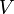$V$ forests of one vertex each, and adds edges one by one, each one causing two trees in the forest to coalesce into one, until all vertices have been placed in the same connected component and the MST is complete. In doing so, one must be careful not to add an edge between two vertices that are already in the same component, for doing so would create a cycle. We shall assume that a spanning tree exists for the following sections. (If you find them too difficult, skip them.)

## Lemma

Suppose that a subset$S$ of the edges of a graph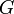$G$ is known to be a subset of the edges of some spanning tree of$G$. Consider the set of edges$T$ containing exactly those edges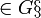$\in G\S$ which, when added to$S$, do not induce a cycle. If a minimal-weight edge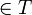$\in T$ is added to$S$, the resulting set is also guaranteed to be a subset of the edges of some spanning tree of$G$. Proof: Consider the graph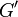$G'$ with vertex set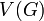$V(G)$ and edge set$S$. Now, any edge in$G\S$ connects either two vertices in different connected components in$G'$ or two vertices in the same component. If it connects two vertices in the same component, adding it generates a cycle (since there is already a path from one component to the other that does not use that edge, and adding the edge generates a return path) and it is not in$T$. Otherwise, it does not generate a cycle because it is a bridge in the resulting single connected component, and it is in$T$. That is,$T$ consists of exactly those edges linking different connected components in$G'$. It is clear that